# The GNU 3DLDF Parabolæ Page

Author: Laurence D. Finston.

This copyright notice applies to the text and source code of this web site, and the graphics that appear on it. The software described in this text has its own copyright notice and license, which can be found in the distribution itself.

Copyright (C) 2005, 2006, 2007 The Free Software Foundation

Permission is granted to copy, distribute, and/or modify this document under the terms of the GNU Free Documentation License, Version 1.2 or any later version published by the Free Software Foundation; with no Invariant Sections, with no Front-Cover Texts, and with no Back-Cover Texts. A copy of this license is included in the file COPYING.TXT

Last updated: April 29, 2006

Back to main page

## Introduction

2005.11.09.
I've now added the data type parabola to the 3DLDF language. It corresponds to the type class Parabola in the C++ code.

The 3DLDF code for generating the following image is in prbla_00.ldf. TeX code for the including it is in prbla_00.txt.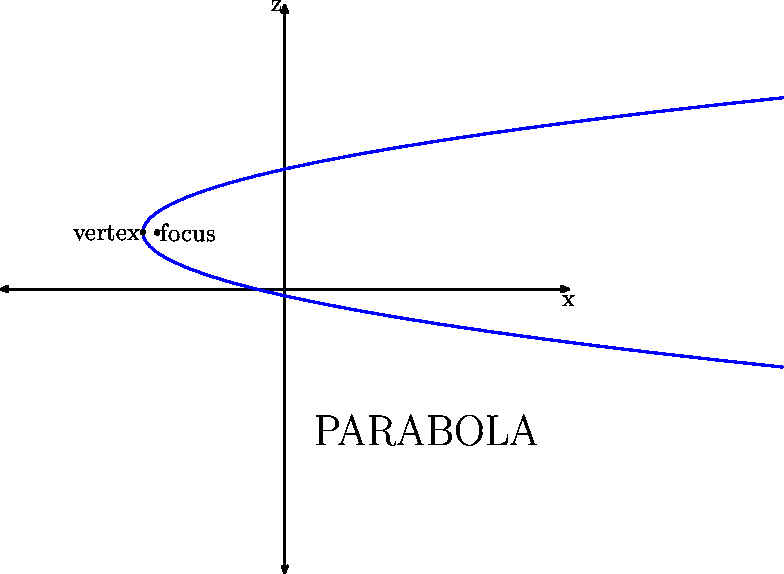Back to contents
Back to main page

## Intersections

### The Intersection of a Parabola and a Linear Path

The 3DLDF code for generating the following image is in prbla_05.ldf. TeX code for the including it is in prbla_05.txt.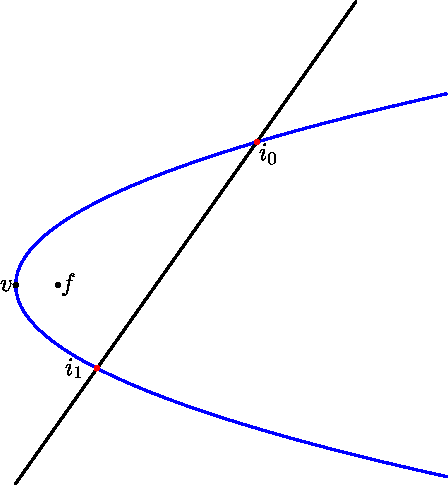The intersection points of a parabola p and a line seqment l such that p and l are coplanar.

The 3DLDF code for generating the following image is in prbla_12.ldf. TeX code for the including it is in prbla_12.txt.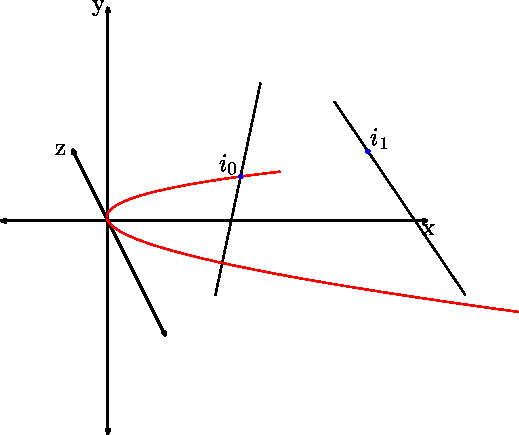The intersection points of a parabola p and two line seqments l and m, such that p and l, and p and m are non-coplanar.

### The Intersection of a Parabola and a Plane

The 3DLDF code for generating the following image can be found in prbla_11.ldf. TeX code for the including it can be found in prbla_11.txt.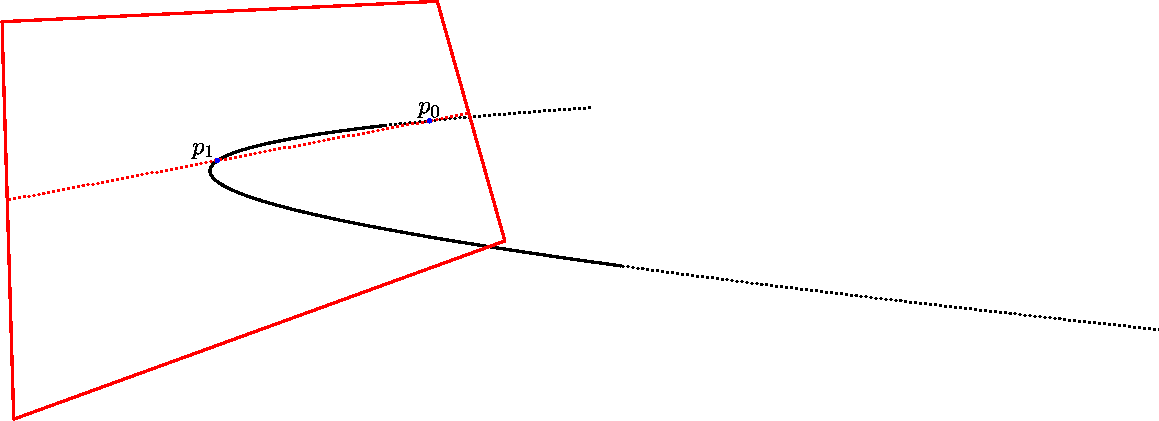The intersection points of a parabola and a plane.

Back to contents
Back to main page

## Pseudo-Paraboloid

The following four images represent a paraboloid generated by rotating a parabola about the x-axis. The circles parallel to the axis of the parabola (the x-axis) are paths created by taking corresponding points from the parabola each time it's rotated.

The 3DLDF code for generating these images is in prbla_01.ldf. TeX code for including it is in prbla_01.txt.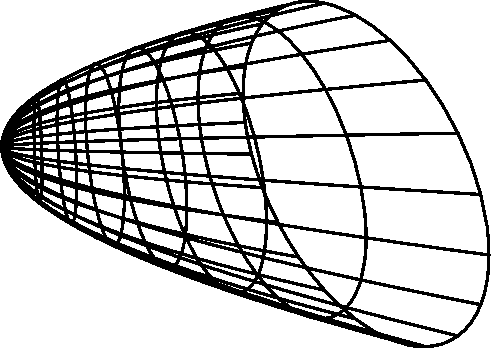Perspective Projection

 Parabola: vertex at origin parameter = 3cm axis = positive x-axis Focus: position: (0, 10cm, -20cm) direction: (0, 10cm, 100cm) distance: 15cm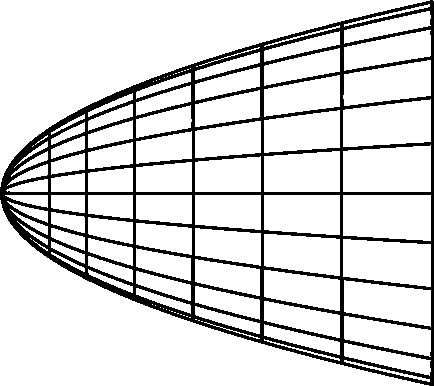Parallel Projection, X-Y PlaneParallel Projection, X-Z Plane

(Similar to the last one, isn't it?)Parallel Projection, Z-Y Plane

Back to contents
Back to main page

## Standardizing

A parabola can be standardized or placed in standard position, i.e., in the x-z plane, with its vertex at the origin and its focus on the positive x-axis.

This is done using the `standardize` operator, which returns a `transform`. `standardize` leaves the `parabola` unchanged. To actually put it into standard position, you must multiply it by the `transform` that was returned.

The following is the gist of the code for creating the following four images. The complete code can be found in prbla_06.ldf. TeX code for including them is in prbla_06.txt.

```parabola p;
set p with_parameter 3 with_extent 7;
rotate p (75, 50);
shift p (3.5, 8, -1.75);
transform t;
t := standardize p;
p *= t;
```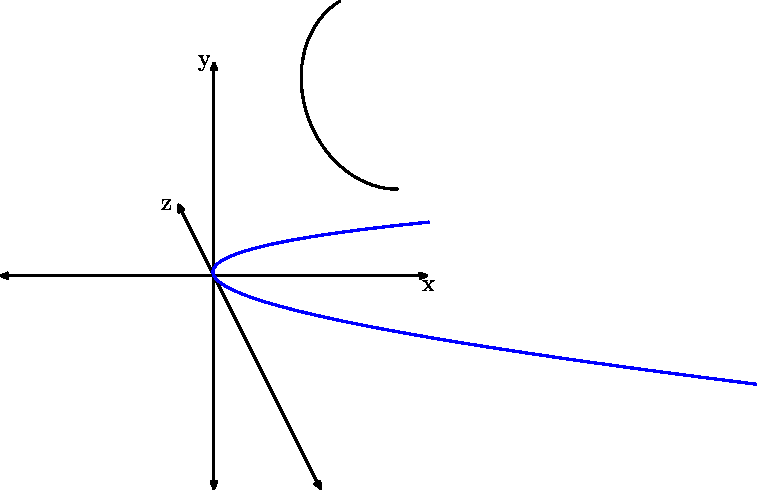Perspective Projection

 Focus: position: (-5cm, 10cm, -20cm) direction: (-5, 10cm, 100cm) distance: 15cmParallel Projection, X-Y Plane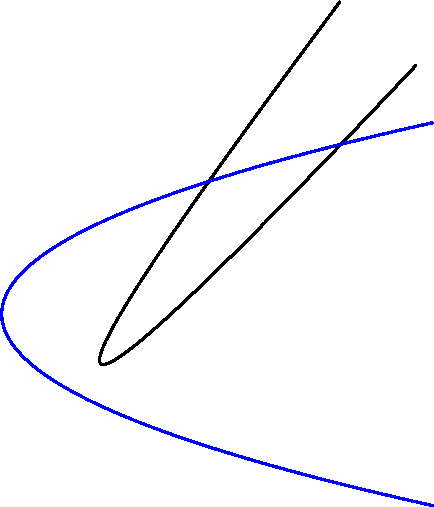Parallel Projection, X-Z PlaneParallel Projection, Z-Y Plane

Back to contents
Back to main page

## Classifying Points with Respect to a Parabola

`points` can be classified according to their position with respect to a `parabola` by using the `location` operator:

```parabola q;
set q with_parameter 3 with_extent 7;
point p;
p := (2, 3, 4.5);
r := p location q;
```

`location` returns one of the following numerical values:

0: The `point` lies on the segment of the parabola represented by the `parabola`.
1: The `point` lies on the parabola, but not the segment, represented by the `parabola` object.
2: The `point` lies in the region enclosed by the branches of the parabola and the line connecting the end points of the segment.
3: The `point` lies between the branches of the parabola, but outside the region enclosed by them and the line connecting the end points of the segment.

-1: The `point` is coplanar with the `parabola`, but does not lie on the curve or between the branches.
-2: The `point` is not coplanar with the `parabola`.
-3: The `parabola` is not parabolic.
-4: The `point` is invalid.
-5: Something has gone terribly wrong.

The complete 3DLDF code for generating the following image can be found in prbla_10.ldf. TeX code for including it can be found in prbla_10.txt.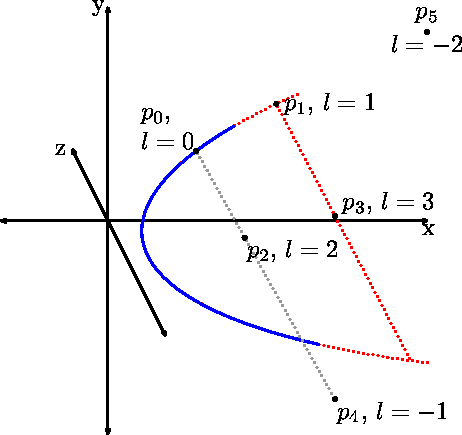Back to contents